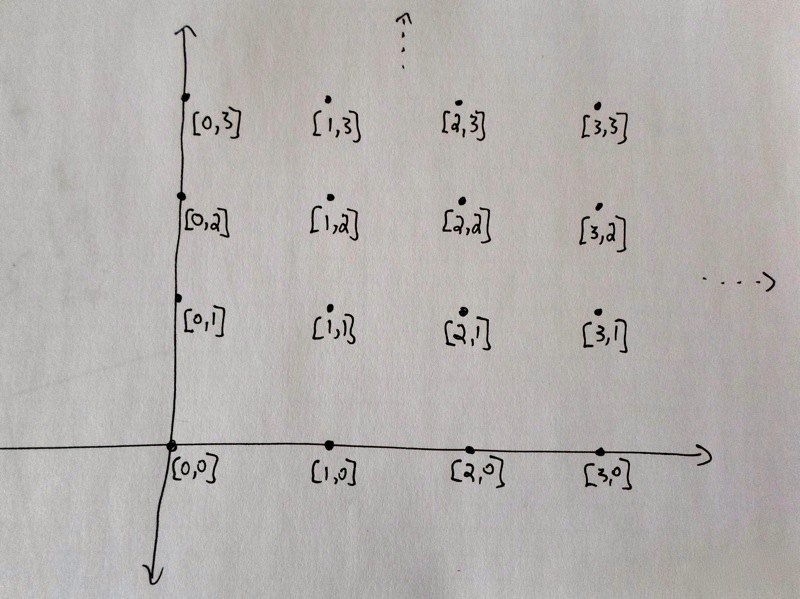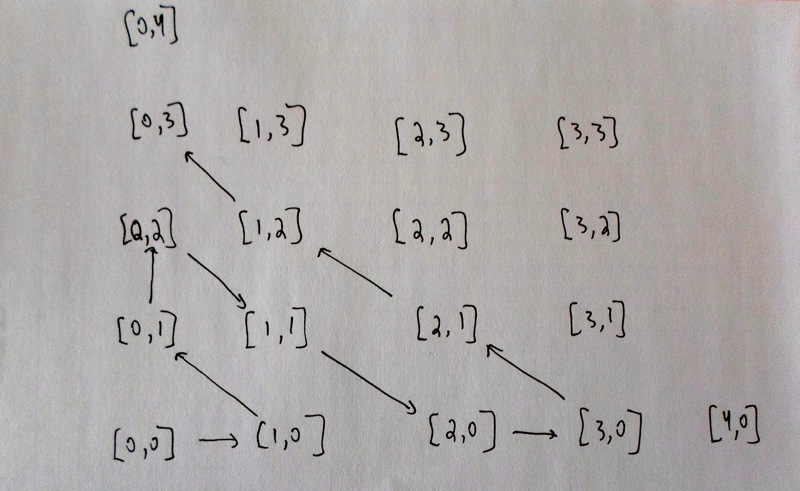# Short

Our goal here is to get a rough idea as to why $$|\mathbb{N}\times\mathbb{N}| = |\mathbb{N}|$$.

In a sense, you could think of this as saying that "infinity times infinity is still just infinity." But this is not very precise, and a key point of this site is that we need to be careful distinguishing different types of infinity.

Recall that $$\mathbb{N}\times\mathbb{N}$$ is the set of pairs of the form $$[a, b]$$, where $$a$$ and $$b$$ are elements of $$\mathbb{N}$$. We can think of it as $$\mathbb{N}$$ copies of $$\mathbb{N}$$.

Remember that to show that $$|\mathbb{N}\times\mathbb{N}| = |\mathbb{N}|$$, we need to construct a one-to-one correspondence between the set $$\mathbb{N}\times\mathbb{N}$$ and the set $$\mathbb{N}$$. In the section on set theory basics, we saw that making a one-to-one correspondence with $$\mathbb{N}$$ is like making a list of elements, so that everything appears exactly once. If we wanted to list all the elements of $$\mathbb{N}\times\mathbb{N}$$, how would we do it?

The key insight is to display the elements of $$\mathbb{N}\times\mathbb{N}$$ in a clever way, and use this to make the list. Remember in the overview we mentioned we can think of this set as a grid on a plane.Now we can start assigning elements of $$\mathbb{N}\times\mathbb{N}$$ in the corner, at $$[0, 0]$$, and working right and up along diagonals. How exactly we do this is somewhat arbitrary, we just have to be sure not to skip anything in $$\mathbb{N}\times\mathbb{N}$$.Then according to this assignment rule, the beginning of our list would look something like the following.

$0 \leftrightarrow [0,0]\\ 1 \leftrightarrow [1, 0]\\ 2 \leftrightarrow [0, 1]\\ 3 \leftrightarrow [0, 2]\\ 4 \leftrightarrow [1, 1]\\ 5 \leftrightarrow [2, 0]\\ 6 \leftrightarrow [3, 0]\\ 7 \leftrightarrow [2, 1]$

By looking at the picture, and the list, we can convince ourselves that every element in $$\mathbb{N}$$ is getting assigned to exactly one element of $$\mathbb{N}\times\mathbb{N}$$, and everything in $$\mathbb{N}\times\mathbb{N}$$ is hit at some point. Therefore we have a one-to-one correspondence! Thus $$|\mathbb{N}\times\mathbb{N}| = |\mathbb{N}|$$.

Top